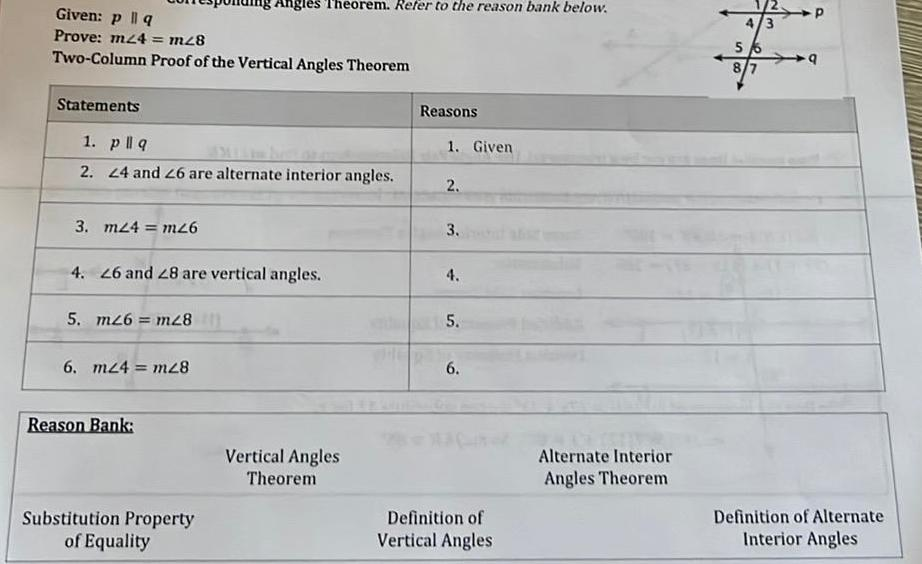Question:

# Given p q Prove m24 m28 Two Column Proof of the Vertical

Last updated: 11/20/2023Given p q Prove m24 m28 Two Column Proof of the Vertical Angles Theorem Statements 1 pllq 2 24 and 26 are alternate interior angles 3 m24 m26 4 26 and 28 are vertical angles 5 m26 m28 Angles Theorem Refer to the reason bank below 6 m24 m28 Reason Bank Substitution Property of Equality Vertical Angles Theorem Reasons THE RE 1 Given 2 3 4 5 6 Definition of Vertical Angles Alternate Interior Angles Theorem 4 3 5 8 7 P 9 Definition of Alternate Interior Angles